Definitions of Square Dance Calls and Concepts

Index -->  Plus  |  A1  |  A2  |  C1  |  C2  |  C3A  |  C3B  |  C4  |  NOL  |
Definitions (Text Only) -->  Plus  |  A1  |  A2  |  C1  |  C2  |  C3A  |  C3B  |  C4  |  NOL  |
 Find call:

Rotate [C1,C2,C3B]
 C1: C2: C3B:Rotates (Rotate, Reverse Rotate, Single Rotate, Reverse Single Rotate) \$B\$O(B, \$B%3!<%k\$r;O\$a\$k(B formation \$B\$K=>\$C\$F(B, \$B0[\$J\$k(B challenge list \$B\$K\$"\$j\$^\$9(B.

C1 \$B\$G\$O(B,
Rotates \$B\$O(B, \$B0lHLE*\$J(B squared set, \$B\$^\$?\$O(B, \$B;w\$?(B formation (\$BNc\$(\$P(B, Column \$B\$N(B Ends \$B\$@\$1(B) \$B\$+\$i\$N\$_%3!<%k\$5\$l\$^\$9(B.
C2 \$B\$G\$O(B,
Rotates \$B\$O(B, \$BJ?9T\$J(B Lines \$B\$^\$?\$O(B 2 x 2 (\$BNc\$(\$P(B, In Your Block Single Rotate 1/4) \$B\$+\$i\$b%3!<%k\$5\$l\$^\$9(B.
C3B \$B\$G\$O(B,
Rotates \$B\$O(B, Columns \$B\$+\$i\$b%3!<%k\$5\$l\$^\$9(B.

Rotates \$B\$rMY\$k\$?\$a\$NJ}K!(B:
1. \$B<+J,\$N(B setup \$B\$NCf?4E@\$r3NG'\$7\$^\$9(B.

\$B<+J,\$N(B setup \$B\$NCf?4E@\$H\$O(B, \$B0J2<\$N>l9g\$r=|\$-(B, square \$B\$N??\$sCf(B (\$B\$h\$/(B "flagpole center (flagpole: \$B4z\$6\$*(B)" \$B\$H8@\$o\$l\$^\$9(B) \$B\$G\$9(B:

1. \$B\$b\$7(B, 2 x 4 formation \$B\$K\$\$\$F(B Lines \$B\$G\$"\$l\$P(B, \$BCf?4E@\$O(B each Box \$B\$N(B Center (\$B\$9\$J\$o\$A(B, Split \$B\$GF0\$-\$^\$9(B) \$B\$G\$9(B. \$B\$b\$7(B, 2 x 4 \$B\$N(B T-Bone \$B\$J\$i(B, Line \$B\$N?M\$,(B Split \$B\$GF0\$/\$N\$KBP\$7\$F(B, Column \$B\$N?M\$NCf?4E@\$O(B flagpole center (squared set \$B\$NCf?4(B) \$B\$G\$9(B.
2. \$B\$b\$7(B, Rotate \$B\$NA0\$K(B Split \$B\$N8@MU\$,\$"\$l\$P(B, formation \$B\$r(B 2 \$B\$D\$NEy\$7\$\$(B subformation \$B\$KJ,\$1\$^\$9(B. \$BCf?4E@\$O(B subformation \$B\$N(B Center \$B\$G\$9(B. 2 x 4 \$B\$+\$i(B, Split \$B\$O(B setup \$B\$r(B 2 \$B\$D\$N(B 2 x 2 \$B\$XJ,\$1\$k\$3\$H\$r0UL#\$7(B, 2 \$B\$D\$N(B 1 x 4 \$B\$G\$O\$"\$j\$^\$;\$s(B.
3. \$B\$b\$7(B, Rotate \$B\$NA0\$K(B, \$BF0\$-\$r9T\$&(B formation \$B\$NL>A0\$,\$"\$l\$P(B, \$BCf?4E@\$O\$=\$N(B formation \$B\$N(B Center \$B\$G\$9(B. \$BNc\$(\$P(B, Columns \$B\$+\$i\$N(B 'Each Column Single Rotate 1/4' \$B\$r9M\$(\$F\$_\$^\$7\$g\$&(B. \$B\$3\$N>l9g(B, \$BCf?4E@\$O(B 1 x 4 setup \$B\$N(B Center \$B\$G\$9(B.
2. \$B2<5-\$N%k!<%k\$K=>\$C\$F(B, \$B3NG'\$7\$?Cf?4E@\$NJ}\$X(B 1/4 \$B8~\$-\$rJQ\$(\$^\$9(B:

 Rotate As Couples 1/4 To Promenade Reverse Rotate As Couples 1/4 To Reverse Promenade Single Rotate Individually 1/4 To Promenade Reverse Single Rotate Individually 1/4 To Reverse Promenade

\$B:8(B\$B8*\$,<+J,\$N(B formation \$B\$N(B Center \$B\$X8~\$/\$h\$&\$K(B 1/4 \$B\$=\$N>l\$G2s\$j\$^\$9(B.
\$B1&(B\$B8*\$,<+J,\$N(B formation \$B\$N(B Center \$B\$X8~\$/\$h\$&\$K(B 1/4 \$B\$=\$N>l\$G2s\$j\$^\$9(B.
3. \$BCf?4E@\$N2s\$j\$G(B Counter Rotate \$B\$rM?\$(\$i\$l\$?(B fraction \$B\$@\$19T\$\$\$^\$9(B.

Squared Set \$B\$+\$i(B, \$B%"%/%F%#%V\$N%@%s%5!<\$O(B set \$B\$N30B&\$r2s\$k\$h\$&\$KF0\$-\$^\$9(B. \$B%"%/%F%#%V\$G\$J\$\$?M\$O(B set \$B\$N(B Center \$B\$XF0\$+\$M\$P\$J\$j\$^\$;\$s(B.

Rotates \$B\$rMY\$k\$H\$-\$K\$O(B, \$B

1. Single \$B\$,\$"\$k\$+\$J\$\$\$+(B;
2. Reverse \$B\$,\$"\$k\$+\$J\$\$\$+(B;
3. \$B\$I\$l\$@\$1F0\$/\$+(B.

C1 Rotates ( Squared Set \$B\$+\$i(B )

Rotate fraction [C1]: (\$B;X<(\$5\$l\$??M\$,(B) \$B%+%C%W%k\$G(B, promenade \$B\$NJ}\$X(B 1/4 \$B8~\$-\$rJQ\$((B; Counter Rotate \$B\$rM?\$(\$i\$l\$?(B fraction \$B\$@\$1(B set \$B\$N30\$r2s\$k\$h\$&\$K9T\$\$(B, \$B;X<(\$5\$l\$J\$+\$C\$??M\$O(B (\$B\$b\$7(B, \$B\$\$\$l\$P(B) Center \$B\$XF0\$-\$^\$9(B.

Reverse Rotate fraction [C1]: (\$B;X<(\$5\$l\$??M\$,(B) \$B%+%C%W%k\$G(B, \$B5U(B promenade \$B\$NJ}\$X(B 1/4 \$B8~\$-\$rJQ\$((B; Counter Rotate \$B\$rM?\$(\$i\$l\$?(B fraction \$B\$@\$1(B set \$B\$N30\$r2s\$k\$h\$&\$K9T\$\$(B, \$B;X<(\$5\$l\$J\$+\$C\$??M\$O(B (\$B\$b\$7(B, \$B\$\$\$l\$P(B) Center \$B\$XF0\$-\$^\$9(B.

Single Rotate fraction [C1]: (\$B;X<(\$5\$l\$??M\$,(B) 1 \$B?M\$G(B, promenade \$B\$NJ}\$X(B 1/4 \$B8~\$-\$rJQ\$((B; Counter Rotate \$B\$rM?\$(\$i\$l\$?(B fraction \$B\$@\$1(B set \$B\$N30\$r2s\$k\$h\$&\$K9T\$\$(B, \$B;X<(\$5\$l\$J\$+\$C\$??M\$O(B (\$B\$b\$7(B, \$B\$\$\$l\$P(B) Center \$B\$XF0\$-\$^\$9(B.

Reverse Single Rotate fraction [C1]: (\$B;X<(\$5\$l\$??M\$,(B) 1 \$B?M\$G(B, \$B5U(B promenade \$B\$NJ}\$X(B 1/4 \$B8~\$-\$rJQ\$((B; Counter Rotate \$B\$rM?\$(\$i\$l\$?(B fraction \$B\$@\$1(B set \$B\$N30\$r2s\$k\$h\$&\$K9T\$\$(B, \$B;X<(\$5\$l\$J\$+\$C\$??M\$O(B (\$B\$b\$7(B, \$B\$\$\$l\$P(B) Center \$B\$XF0\$-\$^\$9(B.

\$BCm
• Rotate \$B\$N8e(B, \$B%3!<%i!<\$N;X<(\$,\$J\$\$8B\$j(B, Face In \$B\$d(B Bend the Line \$B\$r\$7\$J\$\$\$G\$/\$@\$5\$\$(B.
• \$B;X<(\$5\$l\$J\$+\$C\$??M\$O(B, set \$B\$N(B center \$B\$X(B, \$B8~\$-\$rJQ\$(\$:\$KF0\$-\$^\$9(B.
• Rotate 1/4 ( Squared Set \$B\$+\$i(B )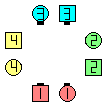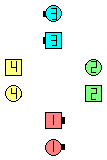Heads Rotate 1/4\$B\$NA0(B As Couples 1/4 To Promenade\$B\$N8e(B Counter Rotate 1/4\$B\$N8e(B (\$B=*\$o\$j(B)
Single Rotate 1/2 ( Squared Set \$B\$+\$i(B )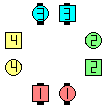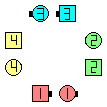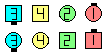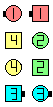Heads Single Rotate 1/2\$B\$NA0(B 1/4 To Promenade\$B\$N8e(B Counter Rotate 1/4\$B\$N8e(B Counter Rotate 1/4 (again)\$B\$N8e(B (\$B=*\$o\$j(B)

C2 Rotates ( \$BJ?9T\$J(B Line \$B\$+\$i(B, \$B\$^\$?\$O(B 2 x 2 (\$B\$?\$@\$7(B Column \$B\$G\$O\$J\$/(B) \$B\$+\$i(B): ):

4 \$B?M\$N(B each Box \$B\$GF0\$-\$^\$9(B.

Rotate fraction[C2]: \$B%+%C%W%k\$G(B, promenade \$B\$NJ}\$X(B 1/4 \$B8~\$-\$rJQ\$((B, (\$B7k2L\$H\$7\$F\$G\$-\$?(B 4 \$B?M\$N(B Line \$B\$GF0\$/\$h\$&\$K(B) Counter Rotate \$B\$rM?\$(\$i\$l\$?(B fraction \$B\$@\$19T\$\$\$^\$9(B. \$B\$3\$l\$O(B, As Couples Zag-Zig \$B\$r\$7\$F(B; Lockit \$B\$r(B n \$B2s\$9\$k\$3\$H\$HF1\$8\$G\$9(B (\$B\$3\$3\$G(B n \$B\$O(B, \$BM?\$(\$i\$l\$?(B fraction \$B\$N(B 1/4 \$B\$N?t(B: 1/4 \$B\$G\$O(B n=1, 1/2 \$B\$G\$O(B n=2, 3/4 \$B\$G\$O(B n=3, etc \$B\$G\$9(B).

Reverse Rotate fraction [C2]: \$B%+%C%W%k\$G(B, \$B5U(B promenade \$B\$NJ}\$X(B 1/4 \$B8~\$-\$rJQ\$((B, (\$B7k2L\$H\$7\$F\$G\$-\$?(B 4 \$B?M\$N(B Line \$B\$GF0\$/\$h\$&\$K(B) Counter Rotate \$B\$rM?\$(\$i\$l\$?(B fraction \$B\$@\$19T\$\$\$^\$9(B. \$B\$3\$l\$O(B, As Couples Zig-Zag \$B\$r\$7\$F(B; Lockit \$B\$r(B n \$B2s\$9\$k\$3\$H\$HF1\$8\$G\$9(B (\$B\$3\$3\$G(B n \$B\$O(B, \$BM?\$(\$i\$l\$?(B fraction \$B\$N(B 1/4 \$B\$N?t\$G\$9(B).

Single Rotate fraction [C2]: 1 \$B?M\$G(B, promenade \$B\$NJ}\$X(B 1/4 \$B8~\$-\$rJQ\$((B, Box Counter Rotate \$B\$rM?\$(\$i\$l\$?(B fraction \$B\$@\$19T\$\$\$^\$9(B. \$B\$3\$l\$O(B, Zag-Zig \$B\$r\$7\$F(B; Box|Split Counter Rotate \$B\$rM?\$(\$i\$l\$?(B fraction \$B\$@\$19T\$&\$3\$H\$HF1\$8\$G\$9(B.

Reverse Single Rotate fraction[C2]: 1 \$B?M\$G(B, \$B5U(B promenade \$B\$NJ}\$X(B 1/4 \$B8~\$-\$rJQ\$((B, Box Counter Rotate \$B\$rM?\$(\$i\$l\$?(B fraction \$B\$@\$19T\$\$\$^\$9(B. \$B\$3\$l\$O(B, Zig-Zag \$B\$r\$7\$F(B; Box|Split Counter Rotate \$B\$rM?\$(\$i\$l\$?(B fraction \$B\$@\$19T\$&\$3\$H\$HF1\$8\$G\$9(B.

Reverse Rotate 1/4 ( \$BJ?9T\$J(B Line \$B\$+\$i(B ):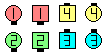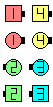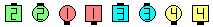Reverse Rotate 1/4\$B\$NA0(B As Couples 1/4 To Reverse Promenade\$B\$N8e(B Line of 4 Counter Rotate 1/4\$B\$N8e(B (\$B=*\$o\$j(B)
Reverse Single Rotate 1/2 ( \$BJ?9T\$J(B Line \$B\$+\$i(B ):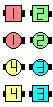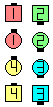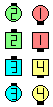Reverse Single Rotate 1/2\$B\$NA0(B 1/4 To Reverse Promenade\$B\$N8e(B Split Counter Rotate 1/2\$B\$N8e(B (\$B=*\$o\$j(B)

Single Rotate 1/4 (Line \$B\$+\$i(B) \$B\$N(B Cheat:
Leaders \$B\$O(B Left-faced U-Turn Back \$B\$r\$7\$F(B; Belles Walk; Beaus Dodge.
Reverse Single Rotate 1/4 (Line \$B\$+\$i(B) \$B\$N(B Cheat:
Leaders \$B\$O(B Right-faced U-Turn Back \$B\$r\$7\$F(B; Beaus Walk; Belles Dodge.

\$BB?\$/\$N%@%s%5!<\$,(B, \$B\$3\$N(B cheat \$B\$r;H\$C\$F\$\$\$k\$H\$\$\$&\$3\$H\$rCN\$C\$F\$*\$\$\$F\$/\$@\$5\$\$(B. \$B\$^\$?(B, \$B7P83\$r\$D\$s\$@%@%s%5!<\$,(B, \$B\$7\$P\$7\$P(B, \$B\$3\$NMM\$KMY\$C\$F\$\$\$k\$h\$&\$K;W\$(\$k\$3\$H\$r8+\$+\$1\$^\$9(B (Trailers \$B\$N\$H\$-\$K(B, \$BC1\$K(B Walk & Dodge \$B\$N\$h\$&\$KF0\$/(B). \$B;dC#\$O(B, 'turn 1/4 & Box Counter Rotate' \$B\$r?4\$NCf\$G9M\$(\$F\$\$\$F(B, flow \$B\$,\$"\$j(B Roll \$B\$G\$-\$k\$H\$\$\$&\$3\$H\$rCN\$C\$F\$\$\$k\$J\$i(B, \$B!V\$h\$7!W\$H\$7\$^\$9(B. \$B\$3\$N(B cheat \$B\$O(B, fraction \$B\$,(B 0/4 \$B\$N\$H\$-\$K\$O;H\$(\$J\$\$\$3\$H\$KCm0U\$7\$F\$/\$@\$5\$\$(B. Fraction \$B\$,(B 1/4 \$B\$h\$jBg\$-\$\$\$H\$-\$K(B, Box Counter Rotates \$B\$,\$"\$j\$^\$9(B.

C3B Rotates ( Column \$B\$H(B, \$BB>\$N(B formation \$B\$+\$i(B ):

Rotate 1/4 ( Column \$B\$+\$i(B ):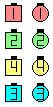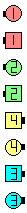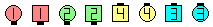Rotate 1/4\$B\$NA0(B As Couples 1/4 To Promenade\$B\$N8e(B Counter Rotate 1/4\$B\$N8e(B (\$B=*\$o\$j(B)
Single Rotate 1/4 ( Column \$B\$+\$i(B ):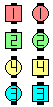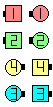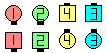Single Rotate 1/4\$B\$NA0(B 1/4 To Promenade\$B\$N8e(B Counter Rotate 1/4\$B\$N8e(B (\$B=*\$o\$j(B)

Single Rotate 1/4 ( T-Bone \$B\$N(B 2 x 4 \$B\$+\$i(B ):

\$B\$3\$NNc\$G\$O(B,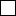\$B\$N?M\$O(B Column \$B\$K\$\$\$k\$H\$7\$FF0\$-(B,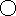\$B\$N?M\$O(B Line \$B\$K\$\$\$k\$H\$7\$FF0\$-(B (\$B\$9\$J\$o\$A(B, Split \$B\$G(B) \$B\$^\$9(B.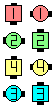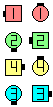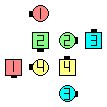before Single Rotate 1/4 after Some work Split: all 1/4 To Promenade Some work Split:all Counter Rotate 1/4 (\$B=*\$o\$j(B)

Split Single Rotate 1/4 ( Column \$B\$+\$i(B ):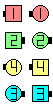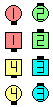before Split Single Rotate 1/4 after Split 1/4 To Promenade after Split Counter Rotate 1/4 (\$B=*\$o\$j(B)

\$BCmSplit Single Rotate \$B\$O(B Single Rotate \$B\$HF1\$8\$G\$9(B.

\$B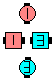\$B\$B;O\$a\$N(B formation: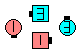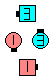after Single Rotate 1/4 after Diamond Single Rotate 1/4 ( See Diamond anything Concept [C4])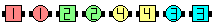\$B\$B;O\$a\$N(B formation: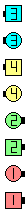Single Rotate 1/4\$B\$N8e(B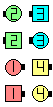Split Single Rotate 1/4\$B\$N8e(B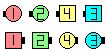Split Split Single Rotate 1/4\$B\$N8e(B

Rotates \$B\$rMY\$k\$?\$a\$N%R%s%H(B:

• Rotate \$B\$^\$?\$O(B Single Rotate \$B\$G\$O(B, \$B:88*(B\$B\$r<+J,\$N(B setup \$B\$N(B center \$B\$NJ}\$XF0\$+\$9\$h\$&\$K(B 1/4 \$B\$=\$N>l\$G2s\$j\$^\$9(B (\$B%+%C%W%k\$+(B 1 \$B?M\$G(B).
• Reverse Rotate \$B\$^\$?\$O(B Reverse Single Rotate \$B\$G\$O(B, \$B1&8*(B\$B\$r<+J,\$N(B setup \$B\$N(B center \$B\$NJ}\$XF0\$+\$9\$h\$&\$K(B 1/4 \$B\$=\$N>l\$G2s\$j\$^\$9(B (\$B%+%C%W%k\$+(B 1 \$B?M\$G(B). ('Reverse' \$B\$H(B 'Right' \$B\$O(B, \$BN>J}(B 'R' \$B\$G;O\$^\$C\$F\$\$\$^\$9(B.)
• Rotate \$B\$O(B \$B!V%+%C%W%k\$G!W(B; Single Rotate \$B\$O(B \$B!V(B1 \$B?M\$G!W(B \$B\$r0UL#\$7\$^\$9(B.
• \$B\$b\$7(B, fraction \$B\$,\$J\$\$\$H\$-\$O(B, 1/4 \$B\$H\$7\$F\$/\$@\$5\$\$(B. \$B;dC#\$N0U8+\$G\$O(B, \$B%3!<%i!<\$O>o\$K(Bfraction \$B\$rM?\$(\$k\$Y\$-\$G\$9(B.
• \$B%3!<%i!<\$O(B fraction 0 (\$B\$^\$?\$O(B 0/4) \$B\$H\$9\$k\$3\$H\$,\$G\$-\$^\$9(B. \$B\$3\$N>l9g(B, \$B\$=\$N>l\$G(B 1/4 \$B2s\$k\$@\$1\$G(B, Counter Rotate \$B\$O2?\$b\$7\$^\$;\$s(B.

\$B\$J\$<(B, Rotate FAMILY \$B\$O(B 3 \$B\$D\$N%l%Y%k\$K\$^\$?\$,\$C\$F\$\$\$k\$N\$G\$7\$g\$&\$+(B? \$BEz\$(\$O(B, Rotate \$B\$NDj5A\$,(B formation \$B\$K0MB8\$7\$F\$\$\$F(B, \$B0l4S@-\$,\$J\$\$\$+\$i\$G\$9(B. \$B;dC#\$N0U8+\$G\$O(B, \$BDj5A\$O%@%s%5!<\$K\$H\$C\$F(B 1 \$B\$D\$N4J7i\$J\$b\$N\$G(B, \$B\$I\$NE,@Z\$J;O\$a\$N(B formation \$B\$+\$i;H\$(\$k\$b\$N\$G\$"\$k\$Y\$-\$G\$9(B. C2 \$B%9%?%\$%k\$N(B Rotates \$B\$K\$OLdBj\$,\$"\$j(B, \$B\$3\$l\$i\$N(B Rotates \$B\$O(B Split Rotate (Rotate \$B\$G\$J\$/(B) \$B\$H(B Split Single Rotate (Single Rotate \$B\$G\$J\$/(B) \$B\$HL>\$E\$1\$k\$Y\$-\$G\$7\$?(B, \$B\$=\$&\$9\$l\$PL7=b\$,\$"\$j\$^\$;\$s(B. \$B;DG0\$J\$,\$i(B, Rotates \$B\$O\$-\$A\$s\$H@0M}\$7\$FDj5A\$5\$l\$^\$;\$s\$G\$7\$?(B.

\$BCounter Rotate [C1].CALLERLAB definition for RotateCALLERLAB definition for Reverse RotateCALLERLAB definition for Single RotateCALLERLAB definition for Reverse Single RotateComments? Questions? Suggestions?

 C1: C2: C3B:https://www.ceder.net/def/rotate_c1.php?level=C1,C2,C3B&language=japan
02-February-2023 10:58:58